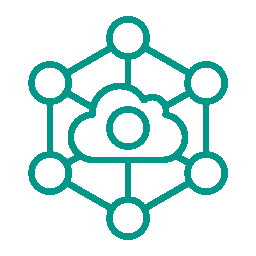•• ARTICLE
• STRING
• CONVERTER
• ENCRYPT
• NETWORK
• MORE
CHART
MATH
• ARTICLE
STRING
CONVERTER
ENCRYPT
NETWORK
MORE
CHART
MATHOnline Tools

# Random Number Generator Online

TAG random

Related Tools
Random Number Generator Online-summary

The online random number generator supports the generation of random integer values and random floating-point values. When unique is specified, all generated random numbers are not repeated.

Random Number Generator Online-instructions

Online random number generator provides online generation of random integers and random floating point numbers (decimals).

1. The download to file function only supports chrome, Firefox and safari browsers.
2. Support integer generation of any size. Support the generation of floating-point numbers of any size, and retain up to 1000 digits after the decimal point.
3. Generator count support[1-10000].
4. The range of generated random numbers is greater than or equal to the minimum value and less than the maximum value.
5. You can specify whether to sort the random number results in More Parameters . Sorting supports unordered, ascending (from small to large) and descending (from large to small). The Result Split can specify the split string of multiple random numbers, such as , generate CSV format data. \n use newline separation. You can also fill in other custom split characters or strings.
6. There are many different ways to generate random numbers. These methods are called random number generators. The most important characteristic of random number is that when it is generated, the latter number has nothing to do with the former number.
7. Real random numbers are generated by using physical phenomena: such as coin tossing, dice, runner, noise using electronic components, nuclear fission, etc. such random number generators are called physical random number generators. Their disadvantage is that they have high technical requirements.
8. In practical applications, it is often enough to use pseudo-random numbers. These series are "seemingly" random numbers. In fact, they are generated by a fixed and repeatable calculation method. Random numbers generated by computers or calculators have a long periodicity. They are not really random because they can actually be calculated, but they have statistical characteristics similar to random numbers. Such a generator is called a pseudo-random number generator. Random numbers generated in general programming languages are pseudo-random numbers.